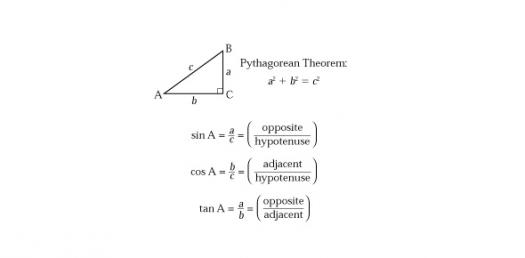# Geometry Questions Of Shape Quiz

23 Questions | Attempts: 329
ShareSettingsIn this quiz you will get angles, shapes, transformations, proofs geometry questions, Let's play!

• 1.
Jonathan has created a new pencil that is in the shape of a triangle. He is trying to develop the best way to market this invention. His pencil seems to be a bit awkward and no sides are the same. He calls it the Scalene Pencil by Big "J". His triangle has side lengths of 3 cm., 1.6 cm., and 2.2 cm. What is the perimeter of his new invention?
• A.

8.6 cm

• B.

6.8 cm.

• C.

6.6 cm.

• D.

4.8 cm.

• 2.
A triangular field has a base of 14 m and a height of 18m. What is the area of the field.
• A.

252 square meters

• B.

64 square meters

• C.

126 square meters

• D.

504 square meters

• 3.
If the base of a rectangle is 25 m and the area is 175 m2, what is the height of the rectangle?
• A.

21,875 m

• B.

100 m

• C.

35 m

• D.

7 m

• 4.
Classify this triangle.  Choose all names that would apply
• A.

Isosceles

• B.

Scalene

• C.

Right

• D.

Obtuse

• E.

Acute

• 5.
Classify the triangle above
• A.

Acute Scalene

• B.

Right Scalene

• C.

Right Isosceles

• D.

Obtuse Sclene

• E.

Acute Isosceles

• 6.
Find the missing angle
• A.

40

• B.

110

• C.

80

• D.

70

• 7.
Classify the triangle.
• A.

Scalene Isosceles

• B.

Acute Scalene

• C.

Acute Isosceles

• D.

Obtuse Scalene

• 8.
A triangle has the following angle measurements:  62 and 57. Classify this triangle by its sides and angles.
• A.

Obtuse Isosceles

• B.

Right Scalene

• C.

Obtuse Scalene

• D.

Acute Scalene

• 9.
A triangle has the following angle measurements:  58 and 73.  What is the missing angle measurement?
• A.

131

• B.

90

• C.

27

• D.

49

• 10.
Choose the classifications that fit the triangle above.
• A.

Obtuse

• B.

Right

• C.

Acute

• D.

Isosceles

• E.

Scalene

• 11.
An equilateral triangle can be classified as an isosceles.
• A.

True

• B.

False

• 12.
Dr. McLaughlin designed a new bulletin board in the shape of a parallelogram. The base of the board is 471.5 cm.,  the height is 21.3 cm., and the side length is 24 cm. What is the perimeter of the McLaughlin B-Board?
• A.

991 cm.

• B.

965.6 cm

• C.

10,316 cm

• D.

10,042.95 cm

• 13.
The area of a trapezoid base 1= 12, base 2= 15, height= 4 is?
• A.

31 units

• B.

60 square units

• C.

35 units

• D.

54 square units

• E.

None of the above

• 14.
Why might you want to measure the area?
• A.

To know how much it will cost me to put carpet in my home

• B.

To know how much grass seed I need for my yard

• C.

All of the above

• D.

None of the above

• 15.
Give all the names that apply to this quadrilateral.
• A.

Parallelogram

• B.

Parallelogram, Rhombus

• C.

Parallelogram, Rectangle

• D.

Parallelogram, Rhombus, Rectangle, Square

• 16.
Give all the names that apply to this quadrilateral.
• A.

Parallelogram

• B.

Parallelogram, Rhombus

• C.

Parallelogram, Rectangle

• D.

Parallelogram, Rhombus, Rectangle, Square

• 17.
Megan wants to build a fence around her vegetable garden. Her garden is 8 ft. long and 3.25 ft wide. How much fencing will Megan need for her wonderful garden?
• A.

26 sq ft

• B.

22.50 ft

• C.

11.25 sq ft

• D.

24 ft

• 18.
What is the height of a rectangle with perimeter 126.6 mm and base length 40.4 mm?**Draw a picture to help**
• A.

86.2 mm

• B.

45.8 mm

• C.

22.9 mm

• D.

5114.64 mm

• 19.
Calculate the area of the figure below
• A.

24

• B.

96

• C.

120

• D.

144

• 20.
Calculate the area of the figure below
• A.

162

• B.

27

• C.

18

• D.

9

• 21.
Find the area of a triangle with a height that is 5 m and half of the base is 9m.
• 22.
Arusha needs to buy fertilizer to spread in her garden. The garden is 20 feet by 15 feet. How much fertilizer will she need to cover her garden?

## Related TopicsBack to top
×

Wait!
Here's an interesting quiz for you.